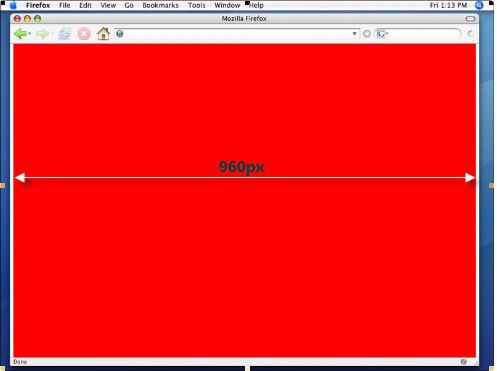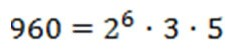### 网站首页页面宽度以多少像素为宜（之一）

##### 当搭建页面结构复杂的门户型网站时，开发工程师们不约而同地都选择将页面宽度定为950px/960px。yahoo 950 淘宝 950 myspace 960 新浪 950 网易 960 live search 958 搜狐 950 优酷 960 aol 960960可以分解为2的6次方乘以3和5, 这使得960可以分割成以下宽度的整数倍：
2, 3, 4, 5, 6, 8, 10, 12, 15, 16, 20, 24, 30, 32, 40, 48, 60, 64, 80, 96, 120, 160, 192, 240, 320, 480

n(960) = n(2^6 * 3 * 5) = 26

 n(480) = n(2^5 * 3 * 5) = 6 * 2 * 2 - 2 = 22 n(750) = n(2 * 3 * 5^3) = 2 * 2 * 4 - 2 = 14 n(800) = n(2^5 * 5^2) = 6 * 3 - 2 = 16 n(1000) = n(2^3 * 5^3) = 4 * 4 - 2 = 14 n(1024) = n(2^10) = 11 - 2 = 9 n(1920) = n(2^7 * 3 * 5) = 8 * 2 * 2 - 2 = 30

n越大，可组合的宽度值就越多。对栅格系统来说，这意味着越灵活！

#### 评论列表

 暂时没有相关记录

#### 发表评论#### 点击排行榜

 【7】网页设计标准尺寸